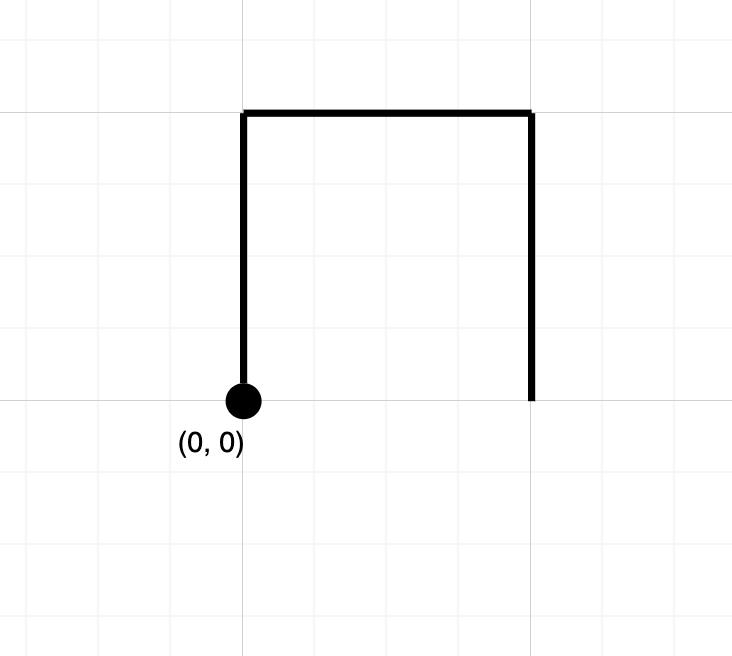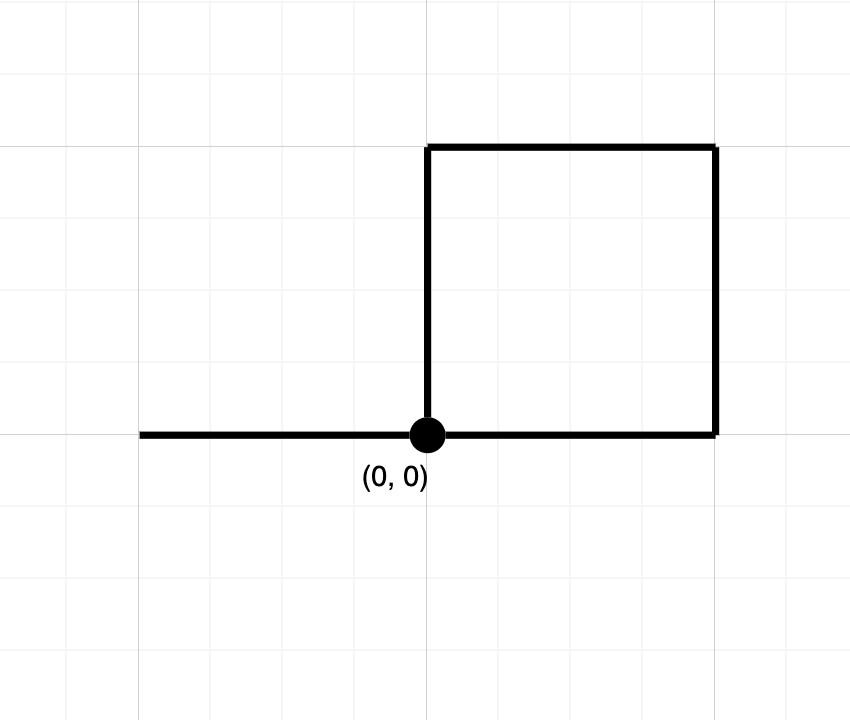# 1496. Path Crossing

Given a string `path`, where `path[i] = 'N'`, `'S'`, `'E'` or `'W'`, each representing moving one unit north, south, east, or west, respectively. You start at the origin `(0, 0)` on a 2D plane and walk on the path specified by `path`.

Return `True` if the path crosses itself at any point, that is, if at any time you are on a location you've previously visited. Return `False` otherwise.

Example 1:```Input: path = "NES"
Output: false
Explanation: Notice that the path doesn't cross any point more than once.
```

Example 2:```Input: path = "NESWW"
Output: true
Explanation: Notice that the path visits the origin twice.```

Constraints:

• `1 <= path.length <= 10^4`
• `path` will only consist of characters in `{'N', 'S', 'E', 'W}`

golang:

``````func isPathCrossing(path string) bool {
if len(path) == 0 {
return true
}

var point = int{0, 0}
var dict = map[int]bool {
point: true,
}
for _, p := range path {
if p == 'N' {
point++
} else if p == 'S' {
point--
} else if p == 'E' {
point++
} else if p == 'W' {
point--
}
if _, ok := dict[point]; ok {
return true
}
dict[point] = true
}

return false
}
``````

Nothing just happens, it's all part of a plan.